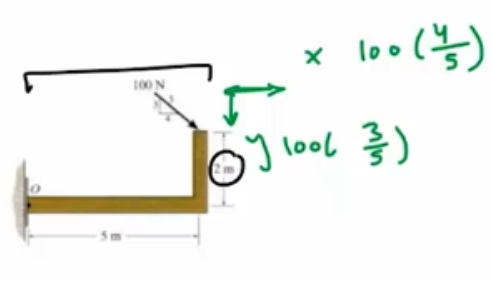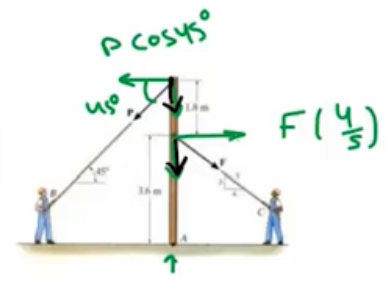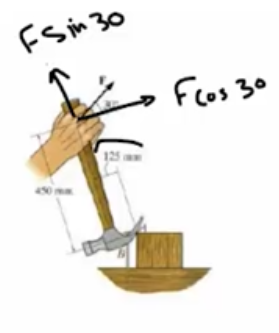Need Help?

Subscribe to Statics

###### \${selected_topic_name}
• Notes

Determine the moment of the force about point $O.$

$\sum M_{0}=100\left(\frac{4}{5}\right)(2)$$+100 \left(\frac{3}{5}\right)(5)$

$=460 \mathrm{N.m}$

If the man at  $B$ exerts a force of  $P=150 \mathrm{N}$ on his , determine the magnitude of the force  $\mathbf{F}$  the man at  $C$  must exert to prevent the pole from rotating, i.e., so the  resultant moment about  $A$ of both forces is zero.

$\sum M_{A}=0$$-F\left(\frac{4}{5}\right)(3 \cdot 6)+P \cos (45)(1.8+3.6)=0$

$∴ F=198 \cdot 8 N$

In order to pull out the nail at  $B,$  the force  $\mathbf{F}$  exerted  on the handle of the hammer must produce a clockwise  moment of  $60 \mathrm{N} \cdot \mathrm{m}$ about point  $A$ .  Determine the required  magnitude of force  $\mathbf{F}$ .

$\sum M_{A}=F \cos 30(0.45)$

$+F \sin 30(0.125)=60$Solver$\longrightarrow$ $F \cos 30(0.45)+F \sin 30(0.125)=60$

$∴ F=132.7 N$